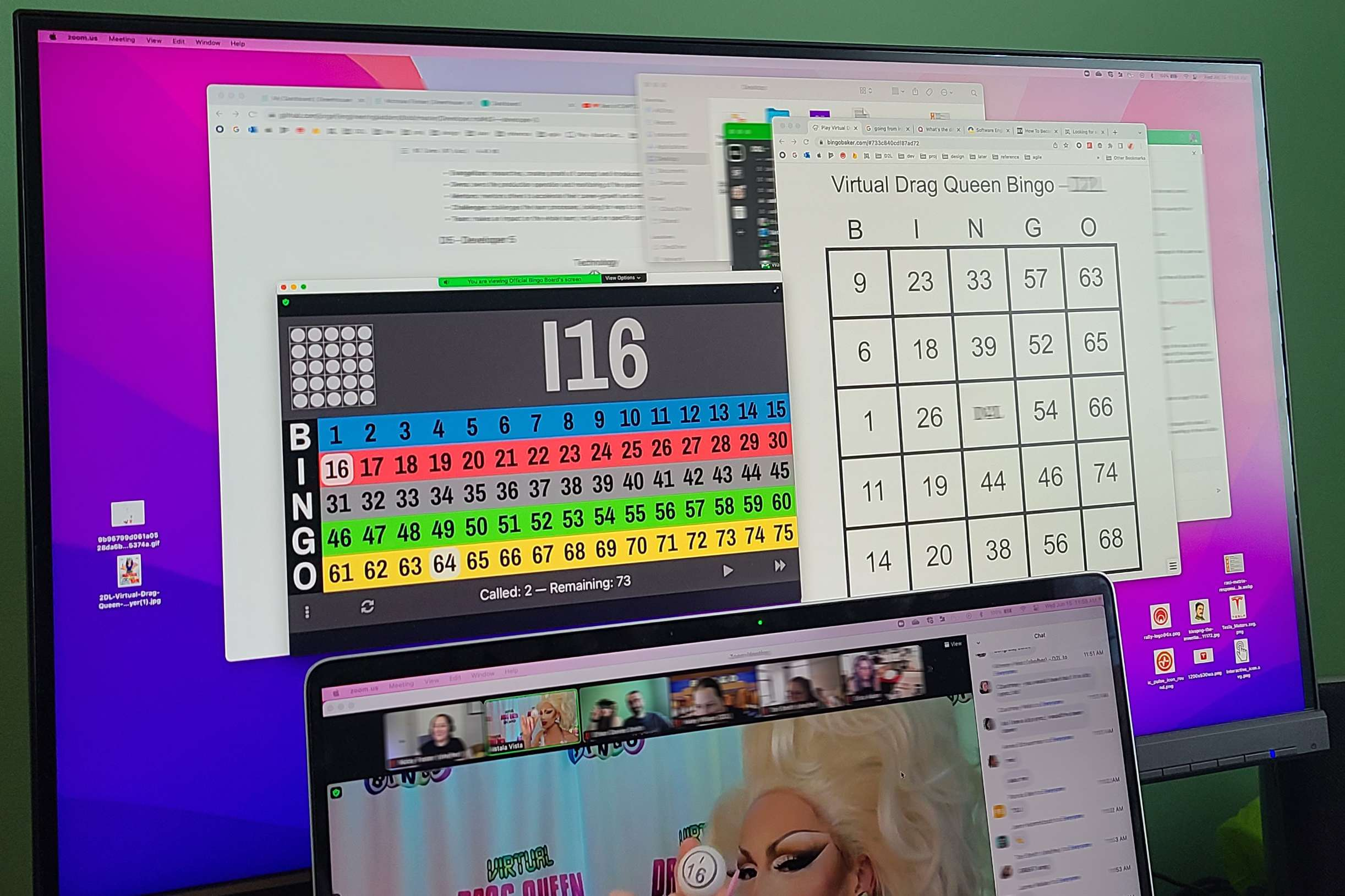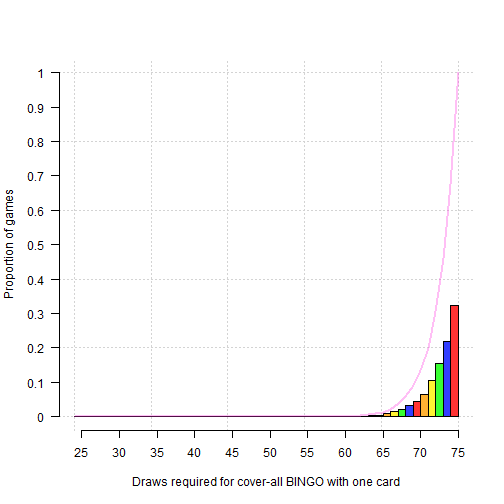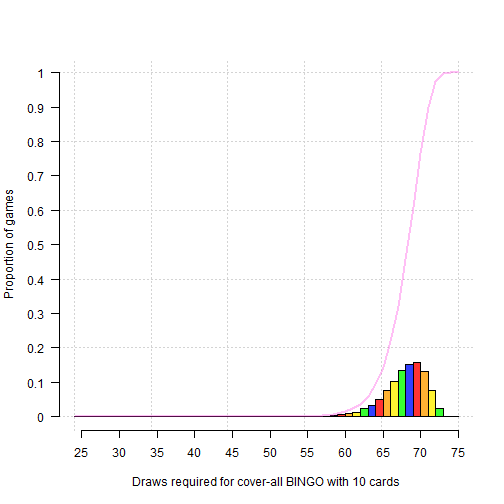# Pride and Probability

To celebrate pride month, my husband Ethan’s workplace Desire2Learn organized virtual Drag Queen BINGO hosted by the fabulous Astala Vista. Even within the confines of a Zoom meeting, Astala Vista put on a great show!

To keep things interesting (and I’m sure to reduce the odds of winning to keep the show going), different BINGO patterns besides the traditional “5 across” BINGO were used. This included a “4 corners” BINGO and a “cover-all” BINGO. To obtain a cover-all BINGO, all numbers on a traditional 5 by 5 BINGO card must be called (noting that of the 25 spaces on the card, 1 is a free space).

Up until this point, probability had not entered the discussion between my husband and I. However, with the cover-all BINGO, Ethan began wondering how many draws it would take to call a cover-all BINGO.I became quiet, and my husband thought he had perhaps annoyed me with all of his probability questions. In fact, I was thinking about how I could easily simulate the answer to his question (and the corresponding combinatorics answer)!

First, we need to randomly generate a BINGO card. A BINGO card features five columns, with five numbers each. The exception is the N column which features a FREE space, given to all players. The B column features the numbers 1 through 15, the I column 16 through 30, etc. The numbers in each column are drawn without replacement for each card.

The following function randomly generates a 5-by-5 BINGO card, with the FREE space taking the value NA.

``````generate_card <- function(){
B <- sample(1:15,  size=5, replace=F)
I <- sample(16:30, size=5, replace=F)
N <- sample(31:45, size=4, replace=F)
G <- sample(46:60, size=5, replace=F)
O <- sample(61:75, size=5, replace=F)

N <- c(N[1:2], NA, N[3:4])
card <- cbind(B, I, N, G, O)
return(card)
}
``````

Here are three examples of BINGO cards generated using our function:

``````card1 <- generate_card()
card2 <- generate_card()
card3 <- generate_card()

cbind(card1, card2, card3)
``````
```##       B  I  N  G  O  B  I  N  G  O  B  I  N  G  O
## [1,]  8 28 44 58 64  7 27 43 46 74  7 28 31 59 74
## [2,]  3 22 37 56 72  8 16 44 59 69  4 19 38 58 62
## [3,] 11 26 NA 49 66  3 28 NA 56 64  1 20 NA 56 72
## [4,] 12 16 35 57 65  4 23 42 51 71 13 30 37 46 68
## [5,]  5 23 43 51 73 14 20 40 47 65  3 25 33 54 70
```

Looks good!

Now, the number of draws required for a cover-all BINGO to occur is obviously dependent on the number of cards used within the BINGO game. Each BINGO card represents only one realization of MANY possible cards. As the number of BINGO cards within a game increases, the number of draws required for a cover-all BINGO should also decrease.

We will begin by assuming there is only a single BINGO card per game.
The following simulation code generates 10,000 BINGO games consisting of 1 card each.

For each BINGO game, the numbers 1 through 75 are sampled without replacement to obtain a draw sequence. A minimum of 24 draws are required for a cover-all BINGO as there are 24 numbers on each BINGO card.

Using a loop, we check whether the numbers on our card are completely contained within the first i=24 drawn numbers. Next, we check if they are contained within the first 25 drawn numbers… and so on and so forth.

When our card is completely contained within the cumulative draw sequence, the loop is broken and the number of draws required for a cover-all BINGO is stored in the vector `draws`.

``````# Set seed for reproducibility!
set.seed(12345)

# Empty vector to store number of draws for each game
draws <- c()

# Simulate 10,000 BINGO games with 1 card each
for (game in 1:10000){

card <- c(generate_card())
draw <- sample(1:75, size=75, replace=F)

for (i in 24:75){
# 24 numbers on card completely contained within cumulative draw sequence?
# if so, break loop and store number of draws
if (sum(card %in% draw[1:i]) == 24){
draws[game] <- i
break
}
}
}
``````

Now, we can estimate the empirical distribution of number of draws required for a cover-all BINGO with a single card based on our 10,000 simulated games.

In the following plot, the height of the rainbow bars represent the proportion of one-card games which required n draws for a cover-all BINGO, where n is the number of draws on the x-axis.

The pink line represents the cumulative probability of winning in n draws. Obviously, we see that 100% of games result in a cover-all BINGO after all possible n=75 draws.

``````rainbow <- c('#FF3333', '#FFB233', '#FFF333', '#39FF33', '#333FFF')

hist(draws, freq=F,
xlab="Draws required for cover-all BINGO with one card",
ylab="Proportion of games",
main="",
breaks=seq(24, 75, 1),
ylim=c(0,1),
xaxt='n', yaxt='n',
col=rainbow,
panel.first=grid())
axis(1, at=seq(25, 75, 5), labels=seq(25, 75, 5))
axis(2, at=seq(0, 1, 0.1), labels=seq(0, 1, 0.1), las=1)

draws_cdf <- ecdf(draws)
lines(24:75, draws_cdf(24:75), col="#FF33E050", lwd=2)
``````Taking a closer look, we see that approximately 32% of all games required 75 draws for a cover-all BINGO. That means for 3,200 games, all 75 balls were drawn before a cover-all BINGO could be declared!

Adding up the probabilities of 56 to 70 draws (0.02 + 0.01 + … + 4.51), only a lucky few games (~13.5% or 1,350 games out of 10,000) required 70 or fewer draws for a BINGO to be declared.

``````# Probability mass function
table(draws) / 10000 * 100
``````
```## draws
##    56    57    58    59    60    61    62    63    64    65    66    67    68    69    70    71    72    73    74    75
##  0.02  0.01  0.01  0.04  0.06  0.09  0.10  0.21  0.32  0.40  0.90  1.59  2.02  3.22  4.51  6.43 10.58 15.37 21.79 32.33
```

Using combinatorics we can compare these empirical probabilities to the true probability. 24 out of 24 numbers on a given card must be drawn to obtain a BINGO. Then, with 70 draws, 70-24=46 of the 75-24=51 numbers not on your card must have been drawn. Finally, the denominator is the number of ways of drawing 70 of 75 BINGO balls uniquely.

The probability of obtaining a cover-all Bingo in 70 or fewer draws with a single card is then:

``````choose(24, 24) * choose(51, 46) / choose(75, 70) * 100
``````
```##  13.61033
```

We see the result of this formula very closely aligns with the 13.5% probability we obtained empirically! Nice.

Now, lets repeat the simulation with 10 cards per game.

``````# Empty vector to store number of draws for each game
draws <- c()

# Simulate 10,000 BINGO games with 1 card each
for (game in 1:10000){

# now generate 10 cards per game
cards <- replicate(n=10, c(generate_card()))
draw <- sample(1:75, size=75, replace=F)

# Empty vector to store number of draws *for each card* within each game
card_draws <- c()
for (c in 1:10){
for (i in 24:75){
# 24 numbers on card completely contained within cumulative draw sequence?
# if so, break loop and store number of draws
if (sum(cards[, c] %in% draw[1:i]) == 24){
card_draws[c] <- i
break
}
}
}
# draws required to win game = min. number of draws across 10 cards
draws[game] <- min(card_draws)
}
``````

Now we see that with 10 cards, no game required 75 draws for a cover-all BINGO!

``````hist(draws, freq=F,
xlab="Draws required for cover-all BINGO with 10 cards",
ylab="Proportion of games",
main="",
breaks=seq(24, 75, 1),
ylim=c(0,1),
xaxt='n', yaxt='n',
col=rainbow,
panel.first=grid())
axis(1, at=seq(25, 75, 5), labels=seq(25, 75, 5))
axis(2, at=seq(0, 1, 0.1), labels=seq(0, 1, 0.1), las=1)

draws_cdf <- ecdf(draws)
lines(24:75, draws_cdf(24:75), col="#FF33E050", lwd=2)
``````Now, ~76% of games (or 7,600 out of 10,000 games) required 70 or fewer draws for a cover-all BINGO!

``````# Probability mass function
table(draws) / 10000 * 100
``````
```## draws
##    50    53    54    55    56    57    58    59    60    61    62    63    64    65    66    67    68    69    70    71    72
##  0.01  0.01  0.01  0.06  0.10  0.14  0.19  0.30  0.64  0.82  1.34  2.27  3.40  5.11  7.53 10.13 13.54 15.31 15.74 13.17  7.69
##    73    74
##  2.27  0.22
```

Probability aside, drag BINGO was a great time and I hope we have the chance to do this again!

# 🌈Wishing all a happy Pride Month wherever you are!!! 🌈